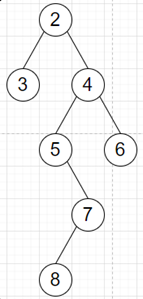# Program to find length of longest alternating path of a binary tree in python

Suppose we have a binary tree, we have to find the longest path that alternates between left and right child and going down.

So, if the input is likethen the output will be 5 as the alternating path is [2, 4, 5, 7, 8].

To solve this, we will follow these steps:

• if root is null, then
• return 0
• Define a function dfs() . This will take node, count, flag
• if node is not null, then
• if flag is same as True, then
• a := dfs(left of node, count + 1, False)
• b := dfs(right of node, 1, True)
• otherwise when flag is same as False, then
• a := dfs(right of node, count + 1, True)
• b := dfs(left of node, 1, False)
• return maximum of a, b
• return count
• From the main method do the following:
• a := dfs(left of root, 1, False)
• b := dfs(right of root, 1, True)
• return maximum of a, b

Let us see the following implementation to get better understanding:

## Example

Live Demo

class TreeNode:
def __init__(self, data, left = None, right = None):
self.data = data
self.left = left
self.right = right

class Solution:
def solve(self, root):
if not root:
return 0

def dfs(node, count, flag):
if node:
if flag == True:
a = dfs(node.left, count + 1, False)
b = dfs(node.right, 1, True)
elif flag == False:
a = dfs(node.right, count + 1, True)
b = dfs(node.left, 1, False)

return max(a, b)
return count

a = dfs(root.left, 1, False)
b = dfs(root.right, 1, True)

return max(a, b)

ob = Solution()
root = TreeNode(2)
root.left = TreeNode(3)
root.right = TreeNode(4)
root.right.left = TreeNode(5)
root.right.right = TreeNode(6)
root.right.left.right = TreeNode(7)
root.right.left.right.left = TreeNode(8)
print(ob.solve(root))

## Input

root = TreeNode(2)
root.left = TreeNode(3)
root.right = TreeNode(4)root.right.left = TreeNode(5)
root.right.right = TreeNode(6)root.right.left.right = TreeNode(7)
root.right.left.right.left = TreeNode(8)

## Output

5